Fenrier Lab

# Fundamentals in Support Vector Machine Algorithm

Let’ talk something about machine learning, it’s inspired by the course of Pro.Andrew Ng.

Consider the following training set which contains elements belong to two different types

$S = \{(x^{(i)}, y_i )\,\mid i = 1,,,n\}$

where $$x^{(i)}$$’s are feature points in d-dimensions, and we use $$y \in \{1,-1\}$$ to denote the class labels. To simplify our discussion, I will use d = 2, and assume that the dataset can be divided into two categories by a straight line, which is also called the separating hyperplane in higher dimensions cases.

$\omega^T x +b = 0$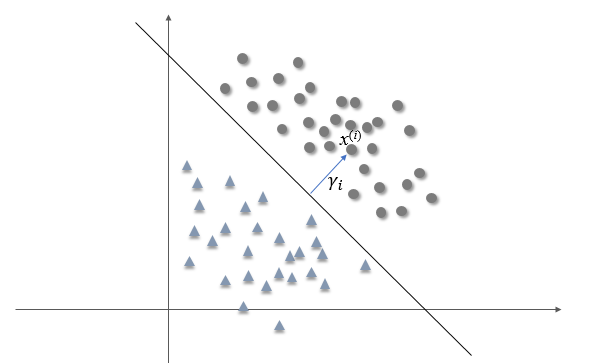The distance from a data point $$x^{(i)}$$ to the hyperplane named margin, and it’s denoted by the symbol $$\gamma_i$$. From 1 to n, we define the margin of the hyperplane with respect to $$S$$ to be smallest of the margins on the individual data points

$\gamma = \min_{i=1 \to n} \quad \gamma_i$

Obviously, given a hyperplane, $$\gamma$$ is a certain value. And our goal in support vector machine is to find a hyperplane which maximize the value of $$\gamma$$, or equivalent to say, the smallest margins of two categories are equal.

$\max_{\omega, b}\,\gamma\\ s.t. \quad \gamma_i \ge \gamma,\quad i =1,2,,,n$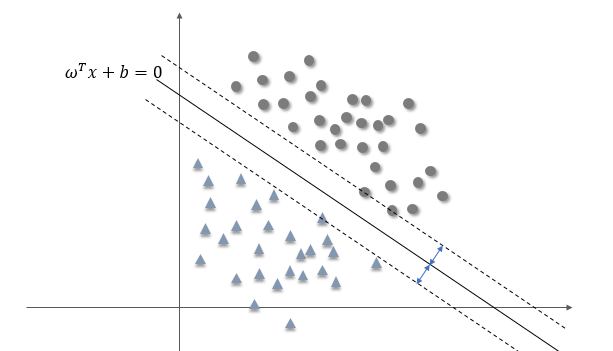To find the value $$\gamma_i$$, let’s consider only one data point as shown in the following to simplify the problem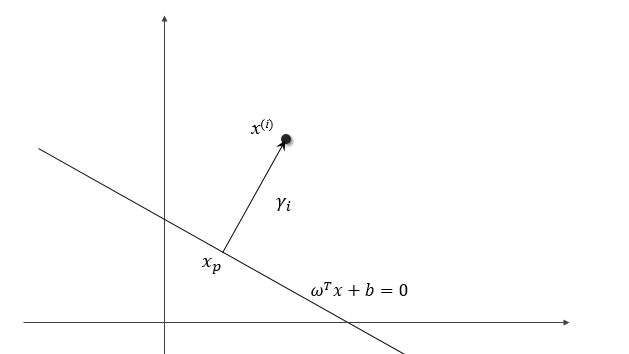Note that $$\omega$$ is orthogonal to the separating hyperplane (it can be proved that this must be the case). We have

$x^{(i)} = x_p + \gamma_i \frac{\omega}{\|\omega\|}$

Since any point lies on the hyperplane must satisfy the equation $$\omega^T x + b = 0$$, hence

\begin{aligned} f(x^{(i)}) &= \omega^T (x_p + \gamma_i \frac{\omega}{\|\omega\|}) + b\\ &= \omega^T x_p + b + \gamma_i \|\omega\|\\ &=\gamma_i \|\omega\|\\ \Rightarrow \gamma_i& =\frac{f(x^{(i)})}{\|\omega\|} =\frac{\omega^T}{\|\omega\|}x^{(i)} + \frac{b}{\|\omega\|} \end{aligned}

Similarly, for the data point on the other side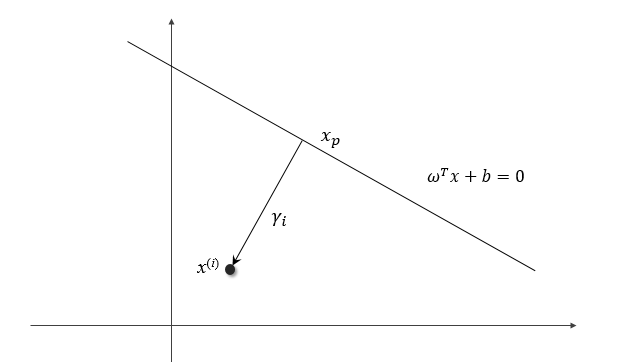We have

$x^{(i)} = x_p - \gamma_i \frac{\omega}{\|\omega\|}$ $\gamma_i =-\left( \frac{\omega^T}{\|\omega\|}x^{(i)} + \frac{b}{\|\omega\|}\right)$

As we have mentioned, suppose $$y_i = 1$$ for the first category, and $$y_i = -1$$ for the another one. Then the margin can be written in a more compact form.

$\gamma_i =y_i \left(\frac{\omega^T}{\|\omega\|}x^{(i)} + \frac{b}{\|\omega\|}\right)$

Then our problem can be written as

$\max_{\omega, b}\,\gamma\\ s.t. \quad y_i \left(\frac{\omega^T}{\|\omega\|}x^{(i)} + \frac{b}{\|\omega\|}\right)\ge \gamma,\quad i =1,2,,,n$

One can multiply on both sides of the inequality constraints condition by $$\|\omega\|$$ simultaneously

$\quad y_i \left(\omega^Tx^{(i)} + b\right) \ge \|\omega\|\gamma\,\,,\, i = 1,,,n$

Note that a hyperplane defined by equation $$m\omega^T x + m b = 0$$ is the same as $$\omega^T x + b = 0$$, but the $$\mathcal{l}^2 -norm$$ of the normal vector of the previous one is $$\|m\omega\| = \sqrt{m}\|\omega\|$$. That means it is our freedom to scale $$\|\omega\|$$ without changing the hyperplane. Let

$\|\omega\| \gamma = 1$

Then we transform our optimization problem into a new formation

$\max_{\omega, b} \frac 1 {\|\omega\|}\\ s.t.\quad g(x^{(i)}) \le 0 ,\, i = 1,,,n$

where $$g(x^{(i)}) =- y_i(\omega^T x^{(i)} + b)+1$$, also it’s equivalent to

$\min_{\omega, b} \frac 1 2 \|\omega\|^2\\ s.t.\quad g(x^{(i)}) \le 0 ,\, i = 1,,,n$

This is a convex optimization problem with inequality constraints, and the method of Lagrange multipliers will be employed. We construct the Lagrangian as

$L(\omega, b,\alpha) = \frac 1 2 \|\omega\|^2 + \sum_{i=1}^n \alpha_i [- y_i(\omega^T x^{(i)} + b)+1]$

where $$\alpha_i$$ ‘s are the Lagrangian multiplier, and they must be non-negative values. Consider the following definition

$\Theta_p(\omega, b) = \max_{\alpha_i \ge 0} L(\omega, b, \alpha)$

As the value of $$\alpha_i$$ can be arbitrarily large, if any of the constrains: $$g(x^{(i)}) \le 0$$ is violated, we are able to make $$\Theta_p(\omega, b) = \infty$$. Conversely, if all of the constrains are to be satisfied, then one can verify that

$\Theta_p(\omega, b) = \frac 1 2 \|\omega\|^2$

Hence, our original optimization problem is also equivalent to

$\min_{\omega, b} \max_{\alpha_i \ge 0} L(\omega, b, \alpha)$

If we allow the the order of $$\min$$ and $$\max$$ to be changed, then we get the formula named Lagrangian dual problem

$\max_{\alpha_i \ge 0}\min_{\omega, b} L(\omega, b, \alpha)$

Generally, the solution of primal problem is always larger than the dual one. However, under certain condition which is named KKT, We claim that

$\min_{\omega, b} \max_{\alpha_i \ge 0} L(\omega, b, \alpha) = \max_{\alpha_i \ge 0}\min_{\omega, b} L(\omega, b, \alpha)$

And the KKT(Karush-Kuhn-Tucker) conditions are shown as following

\begin{aligned} \frac{\partial L}{\partial \omega_i} = 0&, i = 1,,,d \quad(1)\\ \alpha_i g(x^{(i)}) = 0 &,i = 1,,,n \quad (2)\\ g(x^{(i)}) \leq 0&, i = 1,,,n \quad (3) \\ \alpha_i \geq 0&, i=1,,,n \quad (4) \end{aligned}

From equation (2) and (3), we noticed that if $$g(x^{(i)}) < 0$$, then $$\alpha_i= 0$$. And it implies that a large amount of $$\alpha_i$$ ‘s will be 0, since there are many points outside of the two dashed lines which have margin equal to 1 according to the following figure as an example.Before we move on to the continue discussion, let’s have a rest and talk about the non-separable case. More or less, the dataset is not so much “clean”, which means that there may be several “noisy” points and the separating hyperplane is not that good because of the smaller margin, such as the orange line in the following figure. Despite the constraint conditions are violated on few points, the black line seems better than the orange one because of larger minimum margin $$\gamma$$.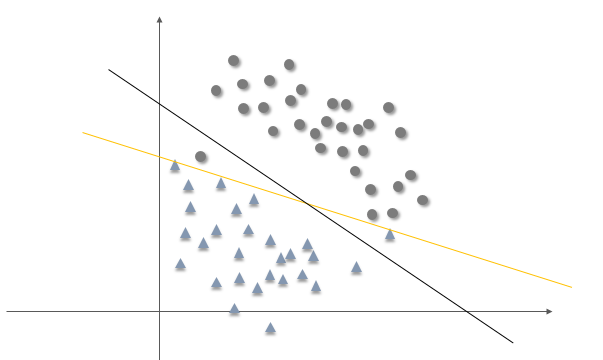To permit the points move cross the hyperplane, We can change the constraint conditions as the following form

$y_i(\omega^T x^{(i)} + b) \ge 1 - \xi_i ,\quad i = 1,,,n, \quad\xi_i \ge 0$

The symbol $$\xi_i$$’s employed by the equations mean that the distance from data points to the hyperplane can be less than one or even zero. But, it makes the constraint conditions non-sense since the value of$$\xi_i$$ can be arbitrarily large. However, this can be avoid by paying a cost of the objective function by adding a value which is proportional to $$\xi_i$$, ie

$\min_{\omega,\xi} \frac 1 2 \|\omega\|^2 + C\sum_{i=1}^n \xi_i \\ s.t.\quad y_i(\omega^T x^{(i)} + b) \ge 1 - \xi_i ,\quad i = 1,,,n\\ \quad\xi_i \ge 0,\quad i = 1,,,n$

And the related Lagrangian function is then modified to

$L(\omega, b, \xi, \alpha, \beta) = \frac 1 2 \|\omega\|^2 + C\sum_{i=1}^n \xi_i + \sum_{i=1}^n \alpha_i [-y_i(\omega^T x^{(i)} + b) + 1 - \xi_i]-\sum_{i=1}^n\beta_i \xi_i$

where $$\beta_i$$’s are additional Lagrangian multipliers, they should also be involved in the dual problem

$\max_{\alpha_i \ge 0,\beta_i\ge 0}\min_{\omega, b, \xi} L(\omega, b,\xi, \alpha,\beta)$

And the KKT condition of $$\beta_i$$ is as following

$\beta_i \xi_i = 0$

First of all, Let’s optimize $$L(\omega, b,\xi, \alpha,\beta)$$ subject to $$\omega, b, \xi$$

$\nabla_\omega L = \omega - \sum_{i=1}^n \alpha_i y_i x^{(i)} = 0$ $\frac{\partial L}{\partial b} = \sum_{i=1}^n \alpha_i y_i = 0$ $\frac{\partial L}{\partial \xi_i} = C -\alpha_i -\beta_i = 0$

and we get

$\omega = \sum_{i=1}^n \alpha_i y_i x^{(i)}\\ \sum_{i=1}^n \alpha_i y_i = 0\\ C =\alpha_i +\beta_i$

Substitute $$\omega, C$$ above into $$L$$, and note that $$\beta_i$$’ are totally determined by $$C$$ and $$\alpha_i$$’s according to $$C =\alpha_i +\beta_i$$, so let the extreme value denoted by $$W(\alpha)$$

\begin{aligned} W(\alpha) &= \frac 1 2 \sum_{i=1}^n \sum_{j=1}^n \alpha_i \alpha_j y_i y_j <x^{(i)}, x^{(j)}> +\sum_{i=1}^n (\alpha_i + \beta_i)\xi_i \\ &-\sum_{i=1}^n \alpha_i y_i\sum_{j=1}^n \alpha_j y_j <x^{(i)}, x^{(j)}> + \sum_{i=1}^n \alpha_i -\sum_{i=1}^n \alpha_i \xi_i - \sum_{i=1}^n \beta_i \xi_i\\ &=-\frac 1 2 \sum_{i=1}^n \sum_{j=1}^n \alpha_i \alpha_j y_i y_j <x^{(i)}, x^{(j)}> + \sum_{i=1}^n \alpha_i \end{aligned}

where $$<x^{(i)}, x^{(j)}>$$ is the inner product of two vector $$x_i$$ and $$x_j$$. It is not hard to see that there is no $$\xi_i$$ or $$\beta_i$$ which is employed to express the non-separable case in $$W(\alpha)$$. Since $$\beta_i \xi_i = 0$$ and $$C =\alpha_i +\beta_i$$, then

$(C - \alpha_i)\xi_i=0$

It means that $$\alpha_i = C$$ so long as $$\xi_i > 0$$, or $$\alpha_i < C$$ when $$\xi_i = 0$$ because $$\beta_i \ge 0$$. And finally, our problem can be converted to the following form

$\max_{\alpha} W(\alpha)\\ s.t.\quad 0 \le \alpha_i \le C,\quad i = 1,,,n\\ \sum_{i=1}^n \alpha_i y_i=0 ,\quad i=1,,,n$